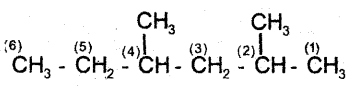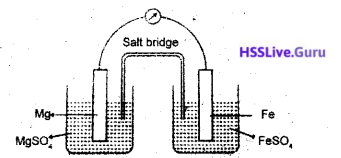# Kerala SSLC Chemistry Model Question Paper 4 English Medium

Students can Download Kerala SSLC Chemistry Model Question Paper 4 English Medium Pdf, Kerala SSLC Chemistry Model Question Papers helps you to revise the complete Kerala State Board New Syllabus and score more marks in your examinations.

## Kerala SSLC Chemistry Model Question Paper 4 English Medium

General Instructions:

1. The first 15 minutes is the cool off time. You may use the time to read and plan your answers.
2. Answer the questions only after reading the instructions and questions thoroughly.
3. Questions with marks series 1, 2, 3 and 4 are categorized as sections A, B, C and D respectively.
4. Five questions are given in each section. Answer any four from each section.
5. Answer each question by keeping the time.

Time: 1½ Hours
Total Score: 40 Marks

Section – A

(Answer any 4 questions from 1 to 5. Each question carries 1 score) (4 × 1 = 4)

Question 1.
The outermost subshell of inert gases except helium
contains electrons. (1)
(2,6,10,14)
6Question 2.
How much is the volume of 32 g of O2 at STP? (1) (Atomic mass O = 16)
22.4 L

Question 3.
The functional group present in organic acids is (1)Question 4.
To which category of medicines does paracetamol belong? (1)
Antipyretic

Question 5.
What is the major constituent of LPG? (1)
ButaneSection – B

(Answer any 4 questions from 6 to 10. Each question carries 2 scores)(4 × 2 = 8)

Question 6.
The order of reactivity of certain metals are as follows:
Mg > Zn > Fe> Cu
a) Which among these does notreactwith dil.HCl?(1)
b) A piece of Mg ribbon is dipped in ZnSO4 solution.
Write down the equation showing the redox reaction taking place. (1)
a) Cu
b) Mg + ZnSO4 → MgSO4 + Zn / Mg + Zn+ → Mg2+ + Zn

Question 7.
Magnetite (Fe3O4), haematite (Fe2O3) and copper pyrites are some ores.
a) Which of the ores is concentrated by froth floatation? (1)
b) Which one is concentrated by magnetic separation? (1)
a) Copper pyrites
b) Magnetite (Fe3O4)Question 8.
Some samples of certain elements are given.a) Which among the above has the highest number of molecules? (1)
b) Which samples occupies a volume of 22.4 L at STP? (1)
[Atomic mass: H=1, He = 4, N = 14 and O = 16]
a) 8g H2
b) 28 g N2

Question 9.
An organic compound has 3 carbon atoms and an OH functional group on the second carbon atom.
a) Give its IUPAC name. (1)
b) Write down the structure of a position isomer of this compound. (1)Question 10.
The third shell of an atomX has 6 electrons.
a) Write the subshell electronic configuration of X. (1)
b) What will be the normal valency of X? (1)
a) 1s22s22p6 3s2 3p4
b) -2

Section – C

(Answer any 4 questions from 11 to 15. Each question carries 3 scores) (4 × 3 = 12)

Question 11.
Cement is an important building material.
a) What are the main raw materials of cement production? (1)
b) What is the role of gypsum in cement manufacute? (1)
c) Cement is not kept in moist places. Why? (1)
a) Limestone, Clay
b) To regulate the setting time of cement
c) Cement absorbs moisture and hardens permanently,

Question 12.
Atomic number of iron is 26.
a) To which group of the periodic table does iron belong? (1)
b) What is the oxidation state of iron in Fe2O3? (1)
c) Write down the subshell electronic configuration of the ion of iron with this oxidation state. (1)
a) 26Fe- 1s22s22p63s23p63d64s2(group-8)
b) +3
c) Fe3+ -1s2 2s2 2p6 3s2 3p6 3d5Question 13.
Consider the equilibrium given
N2O4 + Heat ⇌ 2NO2
a) When does a reversible reaction attain equilibrium? (1)
b) What will happen to the rate of forward reaction if
a high pressure is applied on the system? (1)
(c) At low temperature N2O4 decomposes only slowly. Why? (1)
a) When the speed of forward reaction and backward reaction becomes equal.
b) Rate of foward reaction decreases because due to forward reaction volume (no. of- mols) . increases.
c) Forward reaction is endothermic.,

Question 14.
Complete the following equations:
a) C2H6 + Cl2 → ………+ HCI (1)
b) C3H6 + Cl2 → …………(1)
c) nCH2 = CH2 → …………(1)
a) C2H5Cl
b) C3H6Cl2
c) [CH2 – CH2]nQuestion 15.
The equation showing the reaction of hydrogen with oxygen to form water is given below:
2H2 + O2 → 2H2O
a) What is the ratio of reactant molecules hydrogen and oxygen of the reaction? (1)
b) Suppose 32 g oxygen and 10 g of’hydrogen are allowed to react together.
i) How many molecules of water will be for¬med? (1)
ii) Which reactant will be left behind after the reaction? Hoyv’many moles? (1)
a) 2 : 1
b) i) 2 molecules.
ii) 6 g of H2 will be left behind after the reaction.

Section – D

(Answer any 4 questions from 16 to 20. Each question carries 4 scores)(4 × 4 = 16)

Question 16.
Aluminium is the most abundant metal on the earth’s crust.
a) Name the ore of aluminium. (1)
b) This ore is concentrated by Leaching. What is meant by Leaching? (1)
c) What is the role of cryolite in a aluminium production? (1)
d) Give the name and any one use of an alloy of aluminium (1)
a) Bauxite (Al2O3.2H2O)
b) The ore is dissolved in a suitable solvent and the impurities are filtered out.
c) During the electrolytic refining of aluminium, alumina is dissolved in molten cryolite. Cryolite lowers the melting point of alumina and increase electrical conductivity.
d) Alnico. Used to produce strong magnets.Question 17.Answer the following questions based on the above . structure.
a) Correctly number the chain. CO
b) Name the side chains. (1)
c) Write the IUPAC name of the compound. (1)
d) Give the structure of the straight chain isomer of this hydrocarbon. (1)b) Methyl
c) 2, 4- dimethyl hexane
d) CH3-CH2-CH2-CH2-CH2-CH2-CH2-CH3 (Hectane)

Question 18.
a) How is ethanol manufactured? (2)
b) Complete the equation (1)
CH3 – CH2 – OH + CH3COOH →………….. + H2O
c) To which category of organic compounds does the product of the above reaction belong? (1)
a) Ethanol is manufactured by the fermentation of molasses (sugar solution)
b) CH3 – CH2 – OH + CH3-COOH → CH3 – CO – CH2 – CH3 + H2O
c) EstersQuestion 19.A Galvanic cell is represented above .
a) At which metal electrode does oxidation take place? (1)
b) From which metal to which metal do the electrons flow? (1)
c) Which metal acts as the cathode. (1)
d) Write down the equation showing the redox reaction taking place in the.cell. (1)
(Hint: Reactivity Mg>Fe)
a) Magnessium (Mg)
b) Mg to Fe
c) Iron(Fe)
d)Question 20
Sodium thiosulphate and hydrochloric acid are given.
a) Write an experiment to demonstrate the effect of temperature on reaction rate. (2)
b) What happens to the rate of reaction on increasing the temperature? (1)
c) Write another method to increase the rate of the reaction. (1)